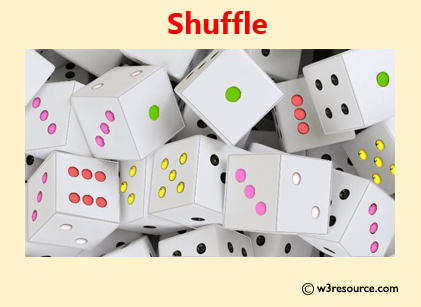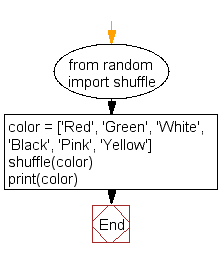﻿ Python: Shuffle and print a specified list - w3resource# Python: Shuffle and print a specified list

## Python List: Exercise - 15 with Solution

Write a Python program to shuffle and print a specified list.Sample Solution:-

Python Code:

``````from random import shuffle
color = ['Red', 'Green', 'White', 'Black', 'Pink', 'Yellow']
shuffle(color)
print(color)
```
```

Sample Output:

```['Yellow', 'Pink', 'Green', 'Red', 'Black', 'White']
```

Flowchart:## Visualize Python code execution:

The following tool visualize what the computer is doing step-by-step as it executes the said program:

Python Code Editor:

Have another way to solve this solution? Contribute your code (and comments) through Disqus.

What is the difficulty level of this exercise?

Test your Python skills with w3resource's quiz

﻿

## Python: Tips of the Day

Checks if the given number falls within the given range.

Example:

```def tips_range(n, start, end = 0):
return start <= n <= end if end >= start else end <= n <= start
print(tips_range(2, 4, 6))
print(tips_range(4, 8))
print(tips_range(1, 3, 5))
print(tips_range(1, 3))
```

Output:

```False
True
False
True
```